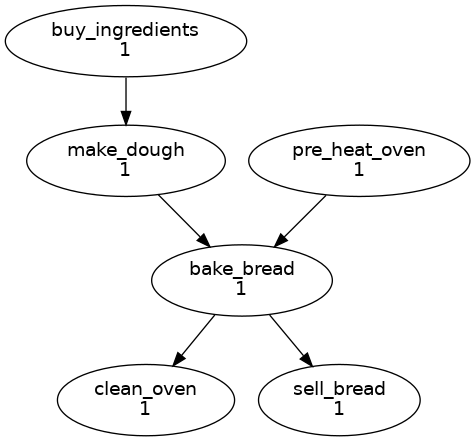# The `flow.cylc` File Format

Aims

You will be able to:
✅ Recognise the `flow.cylc` file format.
✅ Write simple chains of dependencies.

A Cylc workflow is defined by a `flow.cylc` configuration file, which uses a nested INI format:

• Comments start with a `#` character.

• Settings are written as `key = value` pairs.

• Settings can be contained within sections.

• Sections are written inside square brackets i.e. `[section-name]`.

• Sections can be nested, by adding an extra square bracket with each level, so a sub-section would be written `[[sub-section]]`, a sub-sub-section `[[[sub-sub-section]]]`, and so on.

Note

Prior to Cylc 8, `flow.cylc` was named `suite.rc`, but that name is now deprecated.

See Cylc 7 Compatibility Mode for information on compatibility with existing Cylc 7 `suite.rc` files.

## Example

```# Comment
[section]
key = value
[[sub-section]]
another-key = another-value  # Inline comment
yet-another-key = """
A
Multi-line
String
"""
```

## Shorthand

We often use a compact single-line notation to refer to nested config items:

`[section]`

An entire section.

`[section]setting`

A setting within a section.

`[section]setting=value`

The value of a setting within a section.

`[section][sub-section]another-setting`

A setting within a sub-section.

In the file, however, section headings need additional brackets at each level.

## Duplicate Items

Duplicate sections get merged:

 input ```[a] c = C [b] d = D [a] # duplicate e = E ``` result ```[a] c = C e = E [b] d = D ```

Duplicate settings get overwritten:

 input ```a = foo a = bar # duplicate ``` result ```a = bar ```

Except for duplicate graph string items, which get merged:

 input ```R1 = "foo => bar" R1 = "foo => baz" ``` result ```R1 = "foo => bar & baz" ```

## Indentation

It is a good idea to indent `flow.cylc` files for readability.

However, Cylc ignores indentation, so the following examples are equivalent:

 input ```[section] a = A [[sub-section]] b = B b = C # this setting is still # in [[sub-section]] ``` result ```[section] a = A [[sub-section]] b = C ```

## The Dependency Graph

### Graph Strings

Cylc workflows are defined in terms of tasks and dependencies.

Task have names, and dependencies are represented by arrows (`=>`) between them. For example, here’s a task `make_dough` that should run after another task `buy_ingredients` has succeeded:

```buy_ingredients => make_dough
```

These dependencies can be chained together in graph strings:

```buy_ingredients => make_dough => bake_bread => sell_bread
```

Graph strings can be combined to form more complex graphs:

```buy_ingredients => make_dough => bake_bread => sell_bread
```

Graphs can also contain logical operators `&` (and) and `|` (or). For example, the following lines are equivalent to those just above:

```buy_ingredients => make_dough
```

Collectively, all the graph strings make up the workflow dependency graph.

Note

The order of lines in the graph doesn’t matter, so the following examples are equivalent:

 ```foo => bar bar => baz ``` ```bar => baz foo => bar ```

### Cylc Graphs

A non-cycling graph can be defined with `[scheduling][graph]R1`, where `R1` means run once:

```[scheduling]
[[graph]]
R1 = """
"""
```

This is a minimal Cylc workflow that defines a graph of tasks to run, but does not yet say what scripts or applications to run for each task. We will cover that later in the runtime tutorial.

Cylc provides a command line utility for visualising graphs, `cylc graph <path>`, where `path` is the location of the `flow.cylc` file. It generates diagrams similar to the ones you have seen so far. The number `1` below each task is the cycle point. We will explain what this means in the next section.Hint

A graph can be drawn in multiple ways, for instance the following two examples are equivalent:Graphs drawn by `cylc graph` may vary slightly from one run to another, but the tasks and dependencies will always be the same.

Practical

In this practical we will create a new Cylc workflow and write a graph of tasks for it to run.

1. Create a Cylc workflow.

A Cylc workflow is defined by a `flow.cylc` file.

If you don’t have one already, create a `cylc-src` directory in your user space:

```mkdir ~/cylc-src
```

Now create a new workflow source directory called `graph-introduction` under `cylc-src` and move into it:

```mkdir ~/cylc-src/graph-introduction
cd ~/cylc-src/graph-introduction
```

In your new source directory create a `flow.cylc` file and paste the following text into it:

```[scheduler]
[scheduling]
[[graph]]
R1 = """
# Write graph strings here!
"""
```
2. Write a graph.

We now have a blank Cylc workflow. Next we need to define a graph.

Edit your `flow.cylc` file to add graph strings representing the following graph:

3. Visualise the workflow.

Once you have written some graph strings try using `cylc graph` to display the workflow. Run the following command:

```cylc graph .
```

Note

`cylc graph` takes the path to the workflow as an argument. Inside the source directory we can just run `cylc graph .`.

If the results don’t match the diagram above try to correct the graph in your `flow.cylc` file.

Solution

There are multiple correct ways to write this graph. So long as what you see from `cylc graph` matches the above diagram then you have a correct solution.

Two valid examples:

 ```a & c => b => d & f d => e ``` ```a => b => d => e c => b => f ```

The whole workflow should look something like this:

```[scheduler]